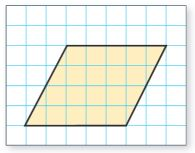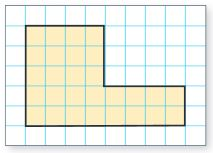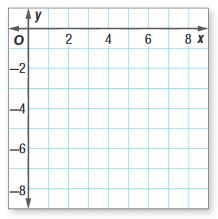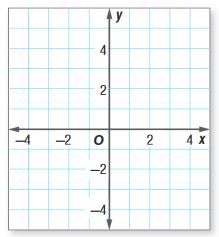Dear guest, you are not a registered member. As a guest, you only have read-only access to our books, tests and other practice materials.

As a registered member you can:

Registration is free and doesn't require any type of payment information. Click here to Register.
Area - Test 4
Keywords: Common Core, Area

Use the coordinates to find the length of each side of the rectangle.
Then find the perimeter.

• Question 1

D(1, 2), E(1, 7), F(4, 7), G(4, 2)

•  Perimeter = units
• Question 2

Q(0, 0), R(4, 0), S(4,4), T(0,4)

•  Perimeter = units
• Question 3

Natasha is building a rectangular picture frame for her favorite photo. The coordinates of the vertices of the frame are (0,0), (0,8), (12, 8), and (12,0). Each grid square has a length of 3 centimeters. Find the amount of wood, in centimeters, needed for the perimeter.

•  Perimeter = cm

Find the area of each figure in square units.

• Question 4•  square units
• Question 5•  square units

Graph each figure and classify it. Then find the area.

• Question 6

R(3, -2), S(7, -2), T(8, -6), V(1, -6)•  Classify: Area: square units
• Question 7

A(-3, -4), B(-3, 5), C(2, 5), D(2, -4)•  Classify: Area: square units

Yes, email page to my online tutor.